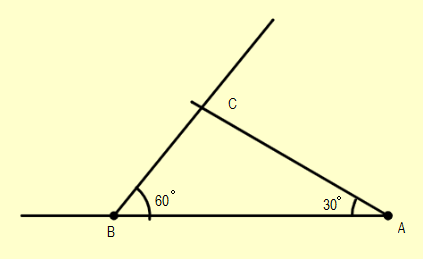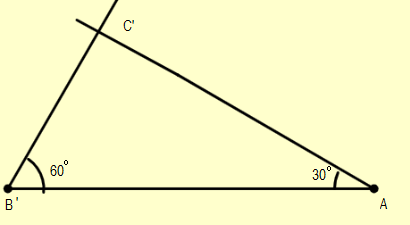QUESTION

# Construct a $\Delta ABC$ in which AB=6cm and $\angle A=30{}^\circ$ and $\angle B=60{}^\circ$ . Construct a $\Delta AB'C'$ similar to $\Delta ABC$ with base AB’=8cm.

Hint: First draw a horizontal line segment of given length followed by drawing another line of $30{}^\circ$ from one point and from another point draw a line at $60{}^\circ$ the lines where cuts gives third point ,join these points we get required triangle.Use the property that two triangles are similar if their corresponding angles are equal and the corresponding sides are in proportion.

It is given that $\Delta ABC\sim \Delta AB'C'$ , and we know two triangles are similar if their corresponding angles are equal and the corresponding sides are in proportion.
AB’=8cm
$\angle A=\angle A=30{}^\circ$
$\angle B=\angle B'=60{}^\circ$

We should first draw a horizontal line segment of length equal to 6 cm and name it as AB, followed by drawing a line at $30{}^\circ$ from the point A and drawing a line at $60{}^\circ$ from the point B. The point where both the lines cut is your point C and the triangle you get is triangle ABC.Now for construction of the $\Delta AB'C'$
We should first draw a horizontal line segment of length equal to 8 cm and name it as AB’, followed by drawing a line at $30{}^\circ$ from the point A and drawing a line at $60{}^\circ$ from the point B’. The point where both the lines cut is your point C’ and the triangle you get is triangle AB’C’.